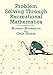Problem Solving Through Recreational Mathematics (Dover Books on Mathematics)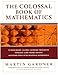The Colossal Book of Mathematics: Classic Puzzles, Paradoxes, and Problems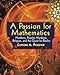A Passion for Mathematics: Numbers, Puzzles, Madness, Religion, and the Quest for Reality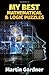My Best Mathematical and Logic Puzzles (Dover Recreational Math)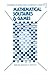Mathematical Solitaires and Games (Excursions in Recreational Mathematics)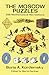The Moscow Puzzles: 359 Mathematical Recreations (Dover Recreational Math)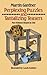Perplexing Puzzles and Tantalizing Teasers (Dover Children's Activity Books)